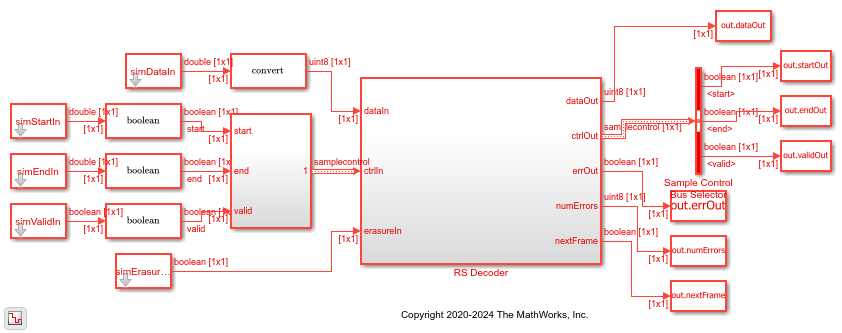# Decode and recover message from RS codeword

This example shows how to use RS Decoder block to decode and recover a message from a Reed-Solomon (RS) codeword. In this example, a set of random inputs are generated and provided to the `comm.RSEncoder` function and its output is provided to the RS Decoder block. The output of the RS Decoder block is compared with the input of the `comm.RSEncoder` function to check whether any errors are encountered. The example model supports HDL code generation for the `RS Decoder` subsystem.

### Set Up Input Data parameters

`Specify the input variables.`
```n = 255; k = 239; primPoly = [1 0 0 0 1 1 1 0 1]; B = 1; nMessages = 4; data = zeros(k,nMessages); inputMsg = (zeros(n,nMessages)); startSig = []; endSig = []; ```

### Generate Random Input Samples

Generate random samples based on n,k, and m values and provide them as input to the `comm.RSEncoder` function. Here, n is the codeword length, k is the message length, and m is the gap between the frames.

```hRSEnc = comm.RSEncoder; hRSEnc.CodewordLength = n; hRSEnc.MessageLength = k; m=0; for ii = 1:nMessages data(:,ii) = randi([0 n],k,1); [inputMsg(1:n,ii)] = hRSEnc(data(:,ii)); inputMsg1(1:n,ii) = inputMsg(1:n,ii); [inputMsg(n+1:n+m,ii)] = zeros(m,1); validIn(1:n,ii) = true; validIn(n+1:n+m) = false; endSig = [endSig [false(n-1,1); true;false(m,1);]]; startSig = [startSig [true;false(n+m-1,1)]]; end refOutput = data(:); ```

### Import Encoded Random Input Samples to the Simulink® Model

Provide the output of the `comm.RSEncoder` function as input to the Simulink model.

```simDataIn = inputMsg(:); simStartIn = startSig(:); simEndIn = endSig(:); simValidIn = validIn(:); ```

Run the Simulink model to export the decoded samples of the Simulink block to the MATLAB® workspace.

```modelname = 'RSDecoder'; open_system(modelname); out = sim(modelname); simOutput = out.dataOut(out.validOut); ```### Compare Simulink Block Output with MATLAB Function Input

Compare the output of the Simulink block with the input provided to the `comm.RSEncoder` function.

```fprintf('\nHDL RS Decoder\n'); difference = double(simOutput) - double(refOutput); fprintf('\nTotal number of samples differed between Simulink block output and MATLAB function output is: %d \n',sum(difference)); ```
```HDL RS Decoder Total number of samples differed between Simulink block output and MATLAB function output is: 0 ```